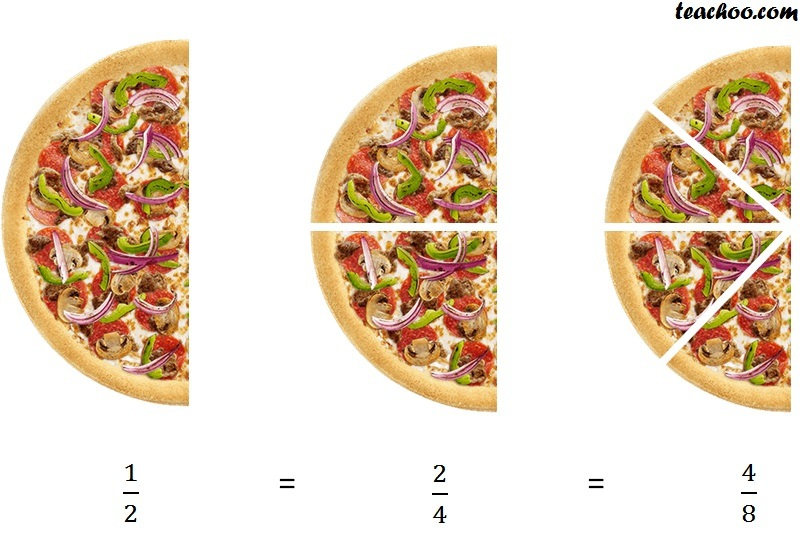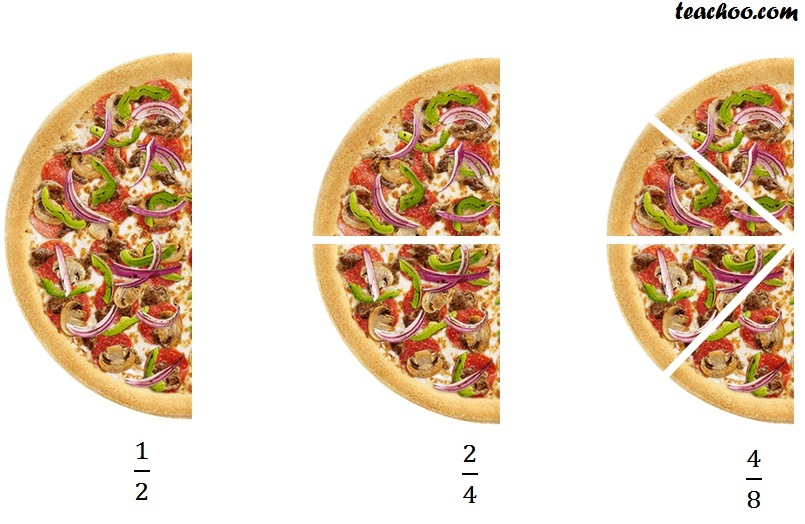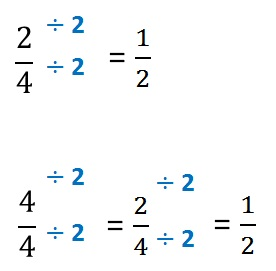Equivalent Fractions

Chapter 7 Class 6 Fractions
Concept wise

Suppose we have a pizza and we eat halfThese fraction look different, but are actually the same.

These are equivalent fractions.

Equivalent fractions are those fractions which look different, but are actually the same.

So, how do we check whether fractions are equivalent?

We simplify them.

Let’s take an example1/2So, 1/2 = 2/4 = 4/8

Here, 1/2 cannot be reduced further.

So, it is the simplest of the fraction.

Learn in your speed, with individual attention - Teachoo Maths 1-on-1 Class

### Transcript

1/2 2/4 4/8 So, 1/2 = 2/4 = 4/8 Here, 1/2 cannot be reduced further. So, it is the simplest of the fraction.